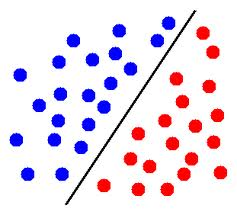# Neural Networks: What does “linearly separable” mean?

0 votes
1 view

I am currently reading the Machine Learning book by Tom Mitchell. When talking about neural networks, Mitchell states:

"Although the perceptron rule finds a successful weight vector when the training examples are linearly separable, it can fail to converge if the examples are not linearly separable. "

I am having problems understanding what he means with "linearly separable"? Wikipedia tells me that "two sets of points in a two-dimensional space are linearly separable if they can be completely separated by a single line."

But how does this apply to the training set for neural networks? How can inputs (or action units) be linearly separable or not?

I'm not the best at geometry and maths - could anybody explain it to me as though I were 5? ;) Thanks!

## 1 Answer

0 votes
by (33.2k points)

Linear separable means that there is a hyperplane

This means that there is a hyperplane, which splits your input data into two half-spaces such that all points of the first class should be in one half-space and other points of the second class should be in the other half-space.

In two dimensional space, it means that there is a line, which separates points of one class from points of the other class.

For example: In the following image, if blue circles represent points from one class and red circles represent points from the other class, then these points are linearly separable.In three dimensions, it means that there is a plane that separates points of one class from points of the other class.

Hope this answer helps.

Visit this Neural Network Tutorial to know more about Neural Network.

0 votes
1 answer
0 votes
1 answer
0 votes
1 answer
0 votes
1 answer
0 votes
1 answer